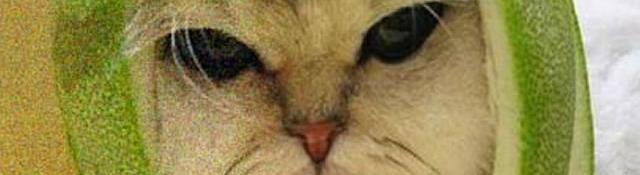## The Technique

Series: Noise reduction by averaging:

1. The Technique
2. The Theory

## What?! Averaging? You mean blurring?

No. This method does not use any type of blurring to reduce noise in a particular image. Infact, the result is the opposite blurring: you get sharp images!

In the averaging method, it is assumed that you have several images of the same object... each with a different "noise pattern". This is exactly what can be easily obtained when taking pictures of distant galaxies. The gigantic telescopes just keep looking at the same object. The noise does affect the images, but at the end of the day, you get multiple images of the object with different "noise patterns"... which is exactly what's needed.

Once you have multiple images, you average them. Yes, the average you learned in school: sum and divide by the total number of images.

You take a coordinate, sum up the value at the position in all images. And divide this sum by the total number of images. We'll look at why this works on the next page. For now, I'll just demonstrate to you this technique using OpenCV.

## Getting ready for the experiment!

Firstly, you need to get multiple pictures... each having some noise. I used photoshop and the Automate option to generate 25 images, each with 8% guassian noise. If you want, you can simply download the 25 pictures below, or generate your own if you wish!Next, create a new project. If you're new to OpenCV, you might want to go through the OpenCV for Beginners article series.

## Juggling code

First, make sure you include the OpenCV library files cv.lib cvaux.lib cxcore.lib highgui.lib. Next, include these headers:

```#include <cv.h>
#include <highgui.h>
```

Now, onto the main function.

```int main()
{
IplImage* imgRed;
IplImage* imgGreen;
IplImage* imgBlue;
```

We begin by declaring three array of 25 image... one for each channel. We'll load the 25 images into these variables now:

```    for(int i=0;i<25;i++)
{
IplImage* img;
char filename;
sprintf(filename, "%d.jpg", (i+1));
imgRed[i] = cvCreateImage(cvGetSize(img), 8, 1);
imgGreen[i] = cvCreateImage(cvGetSize(img), 8, 1);
imgBlue[i] = cvCreateImage(cvGetSize(img), 8, 1);
cvSplit(img, imgRed[i], imgGreen[i], imgBlue[i], NULL);
cvReleaseImage(&img);
}
```

Lets go through the above code line by line. We create a loop (we need to go through 25 images remember?) Inside the loop, we create an image img. This img will temporarily hold an image loaded. Next, we "print into a character string" the name of the file to be loaded. So the variable filename will hold 1.jpg, 2.jpg, etc through the iterations. Then we load the image into img.

Next,we actually allocate memory for the 3 channels of the image loaded. If you don't, you'll end up getting a runtime error. Then, you split img into its constituent channels.

And now that we've split the image, we can safely delete img from the memory to free up some crucial RAM.

Okay, so with that done, we add the following lines:

```    CvSize imgSize = cvGetSize(imgRed);
IplImage* imgResultRed = cvCreateImage(imgSize, 8, 1);
IplImage* imgResultGreen = cvCreateImage(imgSize, 8, 1);

IplImage* imgResultBlue = cvCreateImage(imgSize, 8, 1);

IplImage* imgResult = cvCreateImage(imgSize, 8, 3);
```

These variables hold the result we'll achieve after removing noise by averaging. We're just declaring these variables and allocating memory for them.

Now for the real stuff. Actually doing the averaging:

```    for(int y=0;y<imgSize.height;y++)
{
for(int x=0;x<imgSize.width;x++)
{
int theSumRed=0;
int theSumGreen=0;
int theSumBlue=0;
for(int i=0;i<25;i++)
{
theSumRed+=cvGetReal2D(imgRed[i], y, x);
theSumGreen+=cvGetReal2D(imgGreen[i], y, x);
theSumBlue+=cvGetReal2D(imgBlue[i], y, x);
}
theSumRed = (float)theSumRed/25.0f;
theSumGreen = (float)theSumGreen/25.0f;
theSumBlue = (float)theSumBlue/25.0f;
cvSetReal2D(imgResultRed, y, x, theSumRed);
cvSetReal2D(imgResultGreen, y, x, theSumGreen);
cvSetReal2D(imgResultBlue, y, x, theSumBlue);
}
}
```

We loop through each and every pixel of the image using the first two for loops. Next, we define variables that will hold the sum of the pixels (ie their value) of the 25 different noisy images for each channel.

Then we simply loop through each of the 25 images, getting the value at the current (x, y) and adding it to the respective sum variable.

Once we're done summing up the 25 images, we divide the sum by 25. (hey, we had 25 images!) And then we set the "averaged" value into the result variables!

Finally, once we're done looping through the images, we merge the different channels and display the image:

```    cvMerge(imgResultRed, imgResultGreen, imgResultBlue, NULL, imgResult);
cvNamedWindow("averaged");
cvShowImage("averaged", imgResult);

cvWaitKey(0);

return 0;
}
```

Simple enough right? Here's the result I got from the program:

## Now for the theory

Pretty amazing results right? And this happened with just 25 images. Just imagine the quality of images you'd get if you just sit and take snapshots constantly (like the telescopes do).

Next, we'll take a look at a bit of theory on exactly how this works.

## More in the series

This tutorial is part of a series called Noise reduction by averaging:

1. The Technique
2. The Theory

#### Related posts#### 7 unique neural network architectures